#Function Repository Resource:

# Minimal (1.0.0)current version: 1.1.0 »

Get the minimal item in terms of canonical ordering

Contributed by: Wolfram Staff
 ResourceFunction["Minimal"][list] returns the canonically minimal item in list. ResourceFunction["Minimal"][list,f] uses the ordering function f.

## Details

Values of list are compared using the same canonical order as in Sort.
Use MinimalBy instead to order through a criterion function.
In a numerical list, Ordering[list,n] gives the positions of the n smallest elements. Ordering[list,-n] gives the positions of the n largest elements.
If there are several smallest elements in list, Ordering[list,1] will give only the position of the one that appears first.

## Examples

### Basic Examples (4)

Using canonical ordering, return the minimal item:

 In:=Out=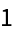Sort the items:

 In:=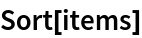Out=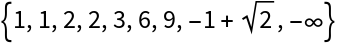The numeric minimum is different:

 In:=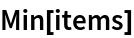Out=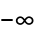Use a different ordering function to obtain the same result:

 In:=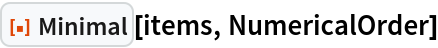Out=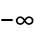### Scope (2)

Use Minimal on an association:

 In:=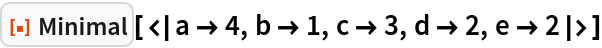Out=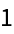Use Minimal on a sparse vector:

 In:=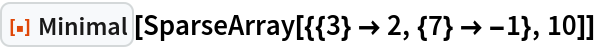Out=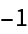### Properties and Relations (2)

Find the item with the smallest second item using an ordering function:

 In:=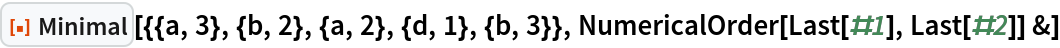Out=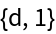The result of the function MinimalBy has a similar form:

 In:=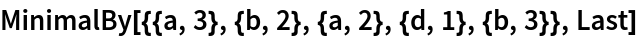Out=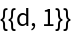## Version History

• 1.1.0 – 30 January 2023
• 1.0.0 – 14 February 2022